# Ohio EOCE Integrated Math I: FOIL & Quadratic Equations Chapter Exam

Exam Instructions:

Choose your answers to the questions and click 'Next' to see the next set of questions. You can skip questions if you would like and come back to them later with the yellow "Go To First Skipped Question" button. When you have completed the practice exam, a green submit button will appear. Click it to see your results. Good luck!

### Page 1

#### Question 3 3. If the equation below told you a ball was 'h' feet in the air after 't' seconds, what would be the first step to finding out when it hit the ground?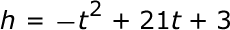### Page 2

#### Question 7 7. Rewrite the following equation in the vertex form.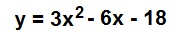#### Question 9 9. Find the roots of the equation.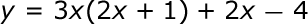### Page 3

#### Question 12 12. When completing the square on the quadratic equation listed below, how is c calculated?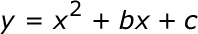#### Question 13 13. What would the first step be in completing the square of the following equation?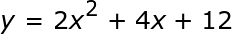#### Question 14 14. Find the value for c and rewrite the equation in the vertex form.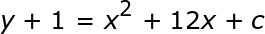### Page 5

#### Question 21 21. Use the quadratic formula to find the solutions to the equation below.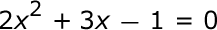### Page 6

#### Question 26 26. Solve the equation below.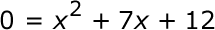#### Question 28 28. Which of the following are the solutions to the equation below?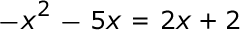#### Ohio EOCE Integrated Math I: FOIL & Quadratic Equations Chapter Exam Instructions

Choose your answers to the questions and click 'Next' to see the next set of questions. You can skip questions if you would like and come back to them later with the yellow "Go To First Skipped Question" button. When you have completed the practice exam, a green submit button will appear. Click it to see your results. Good luck!

Support